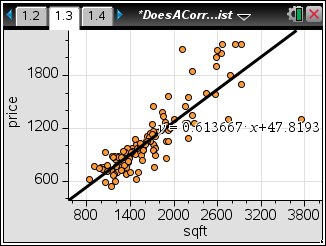### Does a Correlation Exist?

In this activity, students will determine, by examining a graph, if a data set has a positive or negative correlation coefficient. Then, they will find the linear regression equation and calculate the correlation coefficient. They will use this line to predict the value of y for a given x and vice-versa. Finally, students will investigate the effect of outliers and influential points on the regression equation and correlation coefficient.
•TI-Nspire™ CX/CX II
•TI-Nspire™ CX CAS/CX II CAS
• TI-Nspire™ Navigator™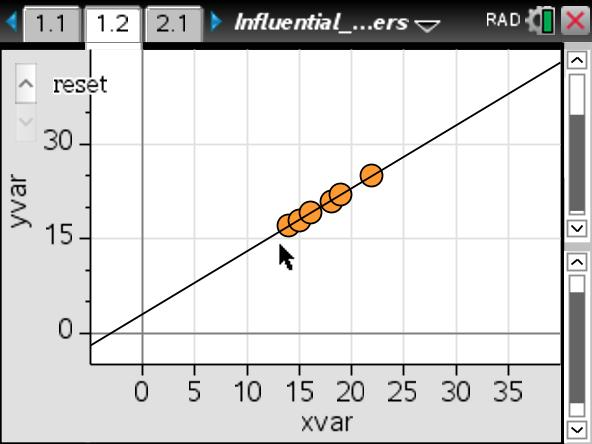### Influence and Outliers

In this activity, students will identify outliers that are influential with respect to the least-squares regression line. Students will describe the role of the location of a point relative to the other data in determining whether that point has influence on the least-squares regression line.
•TI-Nspire™ CX/CX II
•TI-Nspire™ CX CAS/CX II CAS
• TI-Nspire™ Navigator™
• TI-Nspire™
• TI-Nspire™ CAS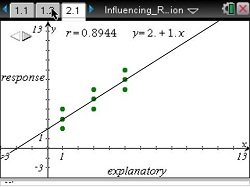### Influencing Regression

This lesson involves a least-squares regression line fit to a set of nine values.
•TI-Nspire™ CX/CX II
•TI-Nspire™ CX CAS/CX II CAS
• TI-Nspire™ Navigator™
• TI-Nspire™
• TI-Nspire™ CAS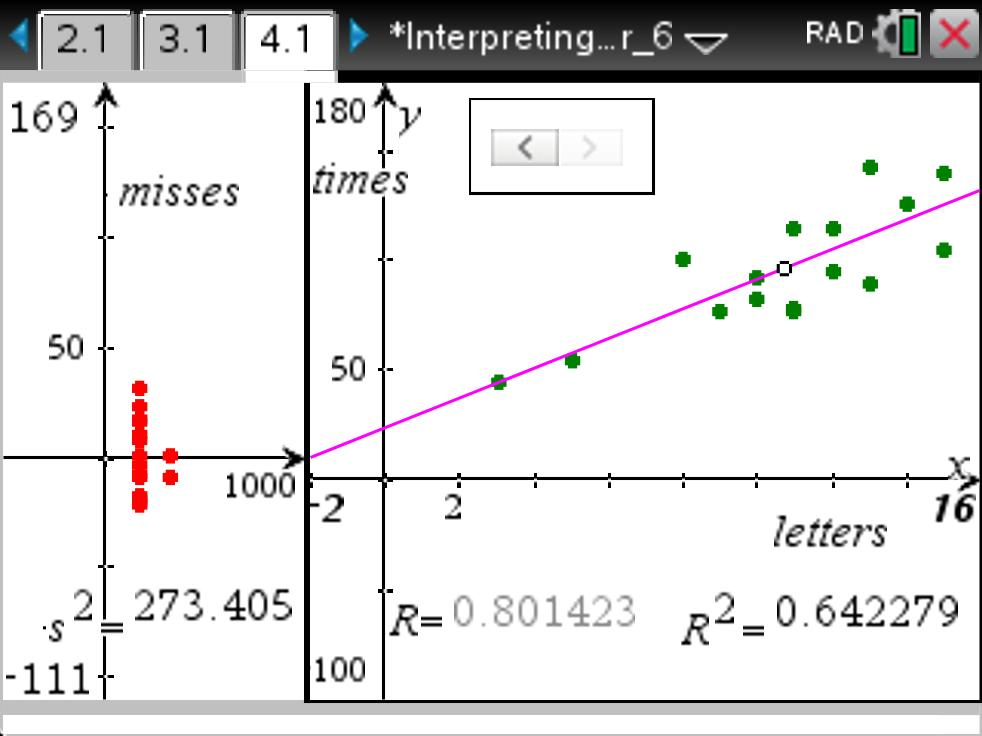### Interpreting R -squared

This lesson involves predicting values of a particular variable.
•TI-Nspire™ CX/CX II
•TI-Nspire™ CX CAS/CX II CAS
• TI-Nspire™ Navigator™
• TI-Nspire™
• TI-Nspire™ CAS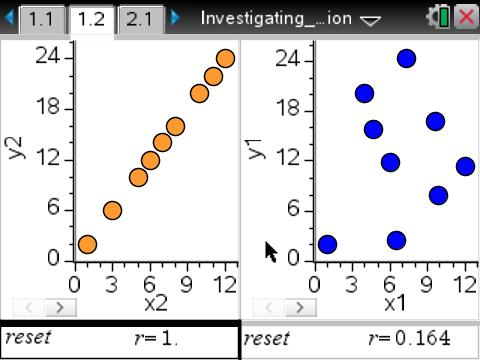### Investigating Correlation

This lesson involves investigating the connection between the scatterplot of bivariate data and the numerical value of the correlation coefficient.
•TI-Nspire™ CX/CX II
•TI-Nspire™ CX CAS/CX II CAS
• TI-Nspire™ Navigator™
• TI-Nspire™
• TI-Nspire™ CAS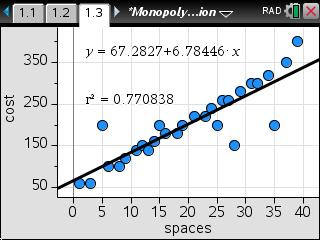### Monopoly and Regression

This lesson involves analyzing the association between the number of spaces from Go and the cost of the property on a standard Monopoly board.
•TI-Nspire™ CX/CX II
•TI-Nspire™ CX CAS/CX II CAS
• TI-Nspire™ Navigator™
• TI-Nspire™
• TI-Nspire™ CAS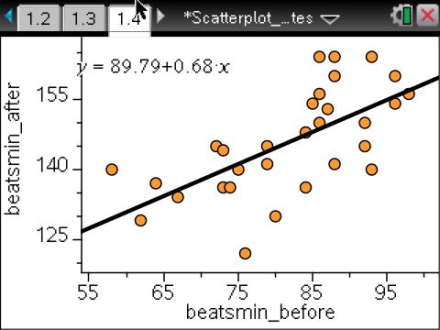### Scatterplot Pulse Rates

This lesson involves creating a scatterplot and fitting a line to student pulse rates collected before and after exercise.
•TI-Nspire™ CX/CX II
•TI-Nspire™ CX CAS/CX II CAS
• TI-Nspire™ Navigator™
• TI-Nspire™
• TI-Nspire™ CAS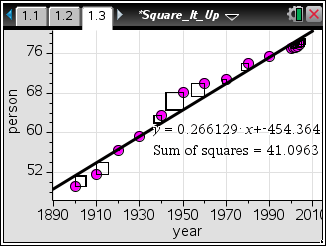### Square it Up!

Students investigate the method of least squares by adding the squares to a scatter plot and moving a line to find the minimum sum. Then they compare their line to the built-in linear regression model.
•TI-Nspire™ CX/CX II
•TI-Nspire™ CX CAS/CX II CAS
• TI-Nspire™ Navigator™
• TI-Nspire™
• TI-Nspire™ CAS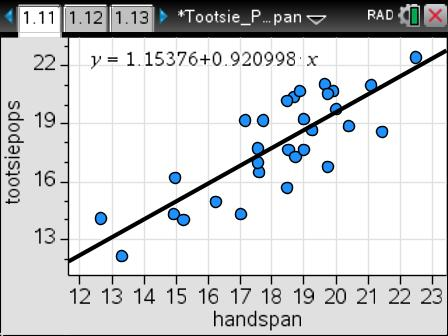### Tootsie Pops & Hand Span

Students will collect data, find the linear regression model of the data, and address aspects of the data that affect regression.
•TI-Nspire™ CX/CX II
•TI-Nspire™ CX CAS/CX II CAS
• TI-Nspire™ Navigator™
• TI-Nspire™
• TI-Nspire™ CAS••